xFunctorEncyclopedia
In category theory
Category theory
Category theory is an area of study in mathematics that examines in an abstract way the properties of particular mathematical concepts, by formalising them as collections of objects and arrows , where these collections satisfy certain basic conditions...

, a branch of mathematics
Mathematics
Mathematics is the study of quantity, space, structure, and change. Mathematicians seek out patterns and formulate new conjectures. Mathematicians resolve the truth or falsity of conjectures by mathematical proofs, which are arguments sufficient to convince other mathematicians of their validity...

, a functor is a special type of mapping between categories. Functors can be thought of as homomorphism
Homomorphism
In abstract algebra, a homomorphism is a structure-preserving map between two algebraic structures . The word homomorphism comes from the Greek language: ὁμός meaning "same" and μορφή meaning "shape".- Definition :The definition of homomorphism depends on the type of algebraic structure under...

s between categories, or morphism
Morphism
In mathematics, a morphism is an abstraction derived from structure-preserving mappings between two mathematical structures. The notion of morphism recurs in much of contemporary mathematics...

s when in the category of small categories
Category of small categories
In mathematics, specifically in category theory, the category of small categories, denoted by Cat, is the category whose objects are all small categories and whose morphisms are functors between categories...

.

Functors were first considered in algebraic topology
Algebraic topology
Algebraic topology is a branch of mathematics which uses tools from abstract algebra to study topological spaces. The basic goal is to find algebraic invariants that classify topological spaces up to homeomorphism, though usually most classify up to homotopy equivalence.Although algebraic topology...

, where algebraic objects (like the fundamental group
Fundamental group
In mathematics, more specifically algebraic topology, the fundamental group is a group associated to any given pointed topological space that provides a way of determining when two paths, starting and ending at a fixed base point, can be continuously deformed into each other...

) are associated to topological space
Topological space
Topological spaces are mathematical structures that allow the formal definition of concepts such as convergence, connectedness, and continuity. They appear in virtually every branch of modern mathematics and are a central unifying notion...

s, and algebraic homomorphisms are associated to continuous
Continuous function
In mathematics, a continuous function is a function for which, intuitively, "small" changes in the input result in "small" changes in the output. Otherwise, a function is said to be "discontinuous". A continuous function with a continuous inverse function is called "bicontinuous".Continuity of...

maps. Nowadays, functors are used throughout modern mathematics to relate various categories. The word functor was borrowed by mathematicians from the philosopher Rudolf Carnap
Rudolf Carnap
Rudolf Carnap was an influential German-born philosopher who was active in Europe before 1935 and in the United States thereafter. He was a major member of the Vienna Circle and an advocate of logical positivism....

, who used the term in a linguistic context.

## Definition

Let C and D be categories
Category (mathematics)
In mathematics, a category is an algebraic structure that comprises "objects" that are linked by "arrows". A category has two basic properties: the ability to compose the arrows associatively and the existence of an identity arrow for each object. A simple example is the category of sets, whose...

. A functor F from C to D is a mapping that
• associates to each object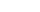an object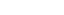,
• associates to each morphism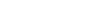a morphism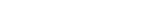such that the following two conditions hold:
•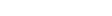for every object•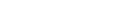for all morphisms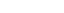and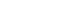That is, functors must preserve identity morphisms and composition of morphisms.

### Covariance and contravariance

There are many constructions in mathematics which would be functors but for the fact that they "turn morphisms around" and "reverse composition". We then define a contravariant functor F from C to D as a mapping that
• associates to each objectan object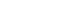• associates to each morphisma morphism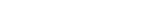such that
•for every object,
•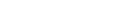for all morphismsandNote that contravariant functors reverse the direction of composition.

Ordinary functors are also called covariant functors in order to distinguish them from contravariant ones. Note that one can also define a contravariant functor as a covariant functor on the dual category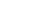. Some authors prefer to write all expressions covariantly. That is, instead of saying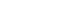is a contravariant functor, they simply write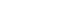(or sometimes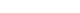) and call it a functor.

Contravariant functors are also occasionally called cofunctors.

### Opposite functor

Every functorinduces the opposite functor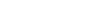, whereand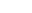are the opposite categories
Opposite category
In category theory, a branch of mathematics, the opposite category or dual category Cop of a given category C is formed by reversing the morphisms, i.e. interchanging the source and target of each morphism. Doing the reversal twice yields the original category, so the opposite of an opposite...

to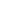and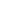. By definition,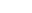maps objects and morphisms identically to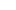. Sincedoes not coincide withas a category, and similarly for,is distinguished from. For example, when composing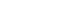with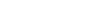, one should use either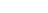or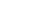. Note that, following the property of opposite category
Opposite category
In category theory, a branch of mathematics, the opposite category or dual category Cop of a given category C is formed by reversing the morphisms, i.e. interchanging the source and target of each morphism. Doing the reversal twice yields the original category, so the opposite of an opposite...

,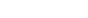.

### Bifunctors and multifunctors

A bifunctor (also known as a binary functor) is a functor in two arguments. The Hom functor
Hom functor
In mathematics, specifically in category theory, hom-sets, i.e. sets of morphisms between objects, give rise to important functors to the category of sets. These functors are called hom-functors and have numerous applications in category theory and other branches of mathematics.-Formal...

is a natural example; it is contravariant in one argument, covariant in the other.

Formally, a bifunctor is a functor whose domain is a product category
Product category
In the mathematical field of category theory, the product of two categories C and D, denoted and called a product category, is a straightforward extension of the concept of the Cartesian product of two sets.-Definition:...

. For example, the Hom functor is of the type Cop × CSet.

A multifunctor is a generalization of the functor concept to n variables. So, for example, a bifunctor is a multifunctor with n = 2.

## Examples

Diagram
Diagram (category theory)
In category theory, a branch of mathematics, a diagram is the categorical analogue of an indexed family in set theory. The primary difference is that in the categorical setting one has morphisms. An indexed family of sets is a collection of sets, indexed by a fixed set; equivalently, a function...

: For categories C and J, a diagram of type J in C is a covariant functor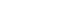.

(Category theoretical) presheaf: For categories C and J, a J-presheaf on C is a contravariant functor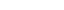.

Presheaves: If X is a topological space
Topological space
Topological spaces are mathematical structures that allow the formal definition of concepts such as convergence, connectedness, and continuity. They appear in virtually every branch of modern mathematics and are a central unifying notion...

, then the open set
Open set
The concept of an open set is fundamental to many areas of mathematics, especially point-set topology and metric topology. Intuitively speaking, a set U is open if any point x in U can be "moved" a small amount in any direction and still be in the set U...

s in X form a partially ordered set
Partially ordered set
In mathematics, especially order theory, a partially ordered set formalizes and generalizes the intuitive concept of an ordering, sequencing, or arrangement of the elements of a set. A poset consists of a set together with a binary relation that indicates that, for certain pairs of elements in the...

Open(X) under inclusion. Like every partially ordered set, Open(X) forms a small category by adding a single arrow UV if and only if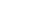. Contravariant functors on Open(X) are called presheaves on X. For instance, by assigning to every open set U the associative algebra
Associative algebra
In mathematics, an associative algebra A is an associative ring that has a compatible structure of a vector space over a certain field K or, more generally, of a module over a commutative ring R...

of real-valued continuous functions on U, one obtains a presheaf of algebras on X.

Constant functor: The functor CD which maps every object of C to a fixed object X in D and every morphism in C to the identity morphism on X. Such a functor is called a constant or selection functor.

Endofunctor: A functor that maps a category to itself.

Identity functor in category C, written 1C or idC, maps an object to itself and a morphism to itself. Identity functor is an endofunctor.

Diagonal functor: The diagonal functor is defined as the functor from D to the functor category DC which sends each object in D to the constant functor at that object.

Limit functor: For a fixed index category J, if every functor JC has a limit
Limit (category theory)
In category theory, a branch of mathematics, the abstract notion of a limit captures the essential properties of universal constructions such as products and inverse limits....

(for instance if C is complete), then the limit functor CJC assigns to each functor its limit. The existence of this functor can be proved by realizing that it is the right-adjoint
In mathematics, the term adjoint applies in several situations. Several of these share a similar formalism: if A is adjoint to B, then there is typically some formula of the type = .Specifically, adjoint may mean:...

to the diagonal functor and invoking the Freyd adjoint functor theorem. This requires a suitable version of the axiom of choice. Similar remarks apply to the colimit functor (which is covariant).

Power sets: The power set functor P : SetSet maps each set to its power set and each function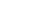to the map which sends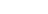to its image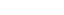. One can also consider the contravariant power set functor which sendsto the map which
sends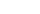to its inverse image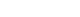Dual vector space: The map which assigns to every vector space
Vector space
A vector space is a mathematical structure formed by a collection of vectors: objects that may be added together and multiplied by numbers, called scalars in this context. Scalars are often taken to be real numbers, but one may also consider vector spaces with scalar multiplication by complex...

its dual space
Dual space
In mathematics, any vector space, V, has a corresponding dual vector space consisting of all linear functionals on V. Dual vector spaces defined on finite-dimensional vector spaces can be used for defining tensors which are studied in tensor algebra...

and to every linear map its dual or transpose is a contravariant functor from the category of all vector spaces over a fixed field
Field (mathematics)
In abstract algebra, a field is a commutative ring whose nonzero elements form a group under multiplication. As such it is an algebraic structure with notions of addition, subtraction, multiplication, and division, satisfying certain axioms...

to itself.

Fundamental group: Consider the category of pointed topological spaces, i.e. topological spaces with distinguished points. The objects are pairs (X, x0), where X is a topological space and x0 is a point in X. A morphism from (X, x0) to (Y, y0) is given by a continuous map f : XY with f(x0) = y0.

To every topological space X with distinguished point x0, one can define the fundamental group
Fundamental group
In mathematics, more specifically algebraic topology, the fundamental group is a group associated to any given pointed topological space that provides a way of determining when two paths, starting and ending at a fixed base point, can be continuously deformed into each other...

based at x0, denoted π1(X, x0). This is the group
Group (mathematics)
In mathematics, a group is an algebraic structure consisting of a set together with an operation that combines any two of its elements to form a third element. To qualify as a group, the set and the operation must satisfy a few conditions called group axioms, namely closure, associativity, identity...

of homotopy
Homotopy
In topology, two continuous functions from one topological space to another are called homotopic if one can be "continuously deformed" into the other, such a deformation being called a homotopy between the two functions...

classes of loops based at x0. If f : XY morphism of pointed space
Pointed space
In mathematics, a pointed space is a topological space X with a distinguished basepoint x0 in X. Maps of pointed spaces are continuous maps preserving basepoints, i.e. a continuous map f : X → Y such that f = y0...

s, then every loop in X with base point x0 can be composed with f to yield a loop in Y with base point y0. This operation is compatible with the homotopy equivalence relation
Equivalence relation
In mathematics, an equivalence relation is a relation that, loosely speaking, partitions a set so that every element of the set is a member of one and only one cell of the partition. Two elements of the set are considered equivalent if and only if they are elements of the same cell...

and the composition of loops, and we get a group homomorphism
Group homomorphism
In mathematics, given two groups and , a group homomorphism from to is a function h : G → H such that for all u and v in G it holds that h = h \cdot h...

from π(X, x0) to π(Y, y0). We thus obtain a functor from the category of pointed topological spaces to the category of groups
Category of groups
In mathematics, the category Grp has the class of all groups for objects and group homomorphisms for morphisms. As such, it is a concrete category...

.

In the category of topological spaces (without distinguished point), one considers homotopy classes of generic curves, but they cannot be composed unless they share an endpoint. Thus one has the fundamental groupoid
Groupoid
In mathematics, especially in category theory and homotopy theory, a groupoid generalises the notion of group in several equivalent ways. A groupoid can be seen as a:...

instead of the fundamental group, and this construction is functorial.

Algebra of continuous functions: a contravariant functor from the category of topological spaces
Topology
Topology is a major area of mathematics concerned with properties that are preserved under continuous deformations of objects, such as deformations that involve stretching, but no tearing or gluing...

(with continuous maps as morphisms) to the category of real associative algebra
Associative algebra
In mathematics, an associative algebra A is an associative ring that has a compatible structure of a vector space over a certain field K or, more generally, of a module over a commutative ring R...

s is given by assigning to every topological space X the algebra C(X) of all real-valued continuous functions on that space. Every continuous map f : XY induces an algebra homomorphism
Algebra homomorphism
A homomorphism between two algebras, A and B, over a field K, is a map F:A\rightarrow B such that for all k in K and x,y in A,* F = kF* F = F + F...

C(f) : C(Y) → C(X) by the rule C(f)(φ) = φ o f for every φ in C(Y).

Tangent and cotangent bundles: The map which sends every differentiable manifold
Differentiable manifold
A differentiable manifold is a type of manifold that is locally similar enough to a linear space to allow one to do calculus. Any manifold can be described by a collection of charts, also known as an atlas. One may then apply ideas from calculus while working within the individual charts, since...

to its tangent bundle
Tangent bundle
In differential geometry, the tangent bundle of a differentiable manifold M is the disjoint unionThe disjoint union assures that for any two points x1 and x2 of manifold M the tangent spaces T1 and T2 have no common vector...

and every smooth map to its derivative
Derivative
In calculus, a branch of mathematics, the derivative is a measure of how a function changes as its input changes. Loosely speaking, a derivative can be thought of as how much one quantity is changing in response to changes in some other quantity; for example, the derivative of the position of a...

is a covariant functor from the category of differentiable manifolds to the category of vector bundle
Vector bundle
In mathematics, a vector bundle is a topological construction that makes precise the idea of a family of vector spaces parameterized by another space X : to every point x of the space X we associate a vector space V in such a way that these vector spaces fit together...

s. Likewise, the map which sends every differentiable manifold to its cotangent bundle
Cotangent bundle
In mathematics, especially differential geometry, the cotangent bundle of a smooth manifold is the vector bundle of all the cotangent spaces at every point in the manifold...

and every smooth map to its pullback is a contravariant functor.

Doing these constructions pointwise gives covariant and contravariant functors from the category of pointed differentiable manifolds to the category of real vector spaces.

Group actions/representations: Every group
Group (mathematics)
In mathematics, a group is an algebraic structure consisting of a set together with an operation that combines any two of its elements to form a third element. To qualify as a group, the set and the operation must satisfy a few conditions called group axioms, namely closure, associativity, identity...

G can be considered as a category with a single object whose morphisms are the elements of G. A functor from G to Set is then nothing but a group action
Group action
In algebra and geometry, a group action is a way of describing symmetries of objects using groups. The essential elements of the object are described by a set, and the symmetries of the object are described by the symmetry group of this set, which consists of bijective transformations of the set...

of G on a particular set, i.e. a G-set. Likewise, a functor from G to the category of vector spaces
Category of vector spaces
In mathematics, especially category theory, the category K-Vect has all vector spaces over a fixed field K as objects and K-linear transformations as morphisms...

, VectK, is a linear representation of G. In general, a functor GC can be considered as an "action" of G on an object in the category C. If C is a group, then this action is a group homomorphism.

Lie algebras: Assigning to every real (complex) Lie group
Lie group
In mathematics, a Lie group is a group which is also a differentiable manifold, with the property that the group operations are compatible with the smooth structure...

its real (complex) Lie algebra
Lie algebra
In mathematics, a Lie algebra is an algebraic structure whose main use is in studying geometric objects such as Lie groups and differentiable manifolds. Lie algebras were introduced to study the concept of infinitesimal transformations. The term "Lie algebra" was introduced by Hermann Weyl in the...

defines a functor.

Tensor products: If C denotes the category of vector spaces over a fixed field, with linear maps as morphisms, then the tensor product
Tensor product
In mathematics, the tensor product, denoted by ⊗, may be applied in different contexts to vectors, matrices, tensors, vector spaces, algebras, topological vector spaces, and modules, among many other structures or objects. In each case the significance of the symbol is the same: the most general...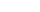defines a functor C × CC which is covariant in both arguments.

Forgetful functors: The functor U : GrpSet which maps a group
Group (mathematics)
In mathematics, a group is an algebraic structure consisting of a set together with an operation that combines any two of its elements to form a third element. To qualify as a group, the set and the operation must satisfy a few conditions called group axioms, namely closure, associativity, identity...

to its underlying set and a group homomorphism
Group homomorphism
In mathematics, given two groups and , a group homomorphism from to is a function h : G → H such that for all u and v in G it holds that h = h \cdot h...

to its underlying function of sets is a functor. Functors like these, which "forget" some structure, are termed forgetful functor
Forgetful functor
In mathematics, in the area of category theory, a forgetful functor is a type of functor. The nomenclature is suggestive of such a functor's behaviour: given some object with structure as input, some or all of the object's structure or properties is 'forgotten' in the output...

s
. Another example is the functor RngAb which maps a ring to its underlying additive abelian group
Abelian group
In abstract algebra, an abelian group, also called a commutative group, is a group in which the result of applying the group operation to two group elements does not depend on their order . Abelian groups generalize the arithmetic of addition of integers...

. Morphisms in Rng (ring homomorphism
Ring homomorphism
In ring theory or abstract algebra, a ring homomorphism is a function between two rings which respects the operations of addition and multiplication....

s) become morphisms in Ab (abelian group homomorphisms).

Free functors: Going in the opposite direction of forgetful functors are free functors. The free functor F : SetGrp sends every set X to the free group
Free group
In mathematics, a group G is called free if there is a subset S of G such that any element of G can be written in one and only one way as a product of finitely many elements of S and their inverses...

generated by X. Functions get mapped to group homomorphisms between free groups. Free constructions exist for many categories based on structured sets. See free object
Free object
In mathematics, the idea of a free object is one of the basic concepts of abstract algebra. It is a part of universal algebra, in the sense that it relates to all types of algebraic structure . It also has a formulation in terms of category theory, although this is in yet more abstract terms....

.

Homomorphism groups: To every pair A, B of abelian groups
Group (mathematics)
In mathematics, a group is an algebraic structure consisting of a set together with an operation that combines any two of its elements to form a third element. To qualify as a group, the set and the operation must satisfy a few conditions called group axioms, namely closure, associativity, identity...

one can assign the abelian group Hom(A,B) consisting of all group homomorphism
Group homomorphism
In mathematics, given two groups and , a group homomorphism from to is a function h : G → H such that for all u and v in G it holds that h = h \cdot h...

s from A to B. This is a functor which is contravariant in the first and covariant in the second argument, i.e. it is a functor Abop × AbAb (where Ab denotes the category of abelian groups
Category of abelian groups
In mathematics, the category Ab has the abelian groups as objects and group homomorphisms as morphisms. This is the prototype of an abelian category....

with group homomorphisms). If f : A1A2 and g : B1B2 are morphisms in Ab, then the group homomorphism Hom(f,g) : Hom(A2,B1) → Hom(A1,B2) is given by φ g o φ o f. See Hom functor
Hom functor
In mathematics, specifically in category theory, hom-sets, i.e. sets of morphisms between objects, give rise to important functors to the category of sets. These functors are called hom-functors and have numerous applications in category theory and other branches of mathematics.-Formal...

.

Representable functors: We can generalize the previous example to any category C. To every pair X, Y of objects in C one can assign the set Hom(X,Y) of morphisms from X to Y. This defines a functor to Set which is contravariant in the first argument and covariant in the second, i.e. it is a functor Cop × CSet. If f : X1X2 and g : Y1Y2 are morphisms in C, then the group homomorphism Hom(f,g) : Hom(X2,Y1) → Hom(X1,Y2) is given by φ g o φ o f.

Functors like these are called representable functor
Representable functor
In mathematics, particularly category theory, a representable functor is a functor of a special form from an arbitrary category into the category of sets. Such functors give representations of an abstract category in terms of known structures In mathematics, particularly category theory, a...

s. An important goal in many settings is to determine whether a given functor is representable.

## Properties

Two important consequences of the functor axiom
Axiom
In traditional logic, an axiom or postulate is a proposition that is not proven or demonstrated but considered either to be self-evident or to define and delimit the realm of analysis. In other words, an axiom is a logical statement that is assumed to be true...

s are:
• F transforms each commutative diagram
Commutative diagram
In mathematics, and especially in category theory, a commutative diagram is a diagram of objects and morphisms such that all directed paths in the diagram with the same start and endpoints lead to the same result by composition...

in C into a commutative diagram in D;
• if f is an isomorphism
Isomorphism
In abstract algebra, an isomorphism is a mapping between objects that shows a relationship between two properties or operations.  If there exists an isomorphism between two structures, the two structures are said to be isomorphic.  In a certain sense, isomorphic structures are...

in C, then F(f) is an isomorphism in D.

One can compose functors, i.e. if F is a functor from A to B and G is a functor from B to C then one can form the composite functor G∘F from A to C. Composition of functors is associative where defined. Identity of composition of functors is identity functor. This shows that functors can be considered as morphisms in categories of categories, for example in the category of small categories
Category of small categories
In mathematics, specifically in category theory, the category of small categories, denoted by Cat, is the category whose objects are all small categories and whose morphisms are functors between categories...

.

A small category with a single object is the same thing as a monoid
Monoid
In abstract algebra, a branch of mathematics, a monoid is an algebraic structure with a single associative binary operation and an identity element. Monoids are studied in semigroup theory as they are naturally semigroups with identity. Monoids occur in several branches of mathematics; for...

: the morphisms of a one-object category can be thought of as elements of the monoid, and composition in the category is thought of as the monoid operation. Functors between one-object categories correspond to monoid homomorphism
Homomorphism
In abstract algebra, a homomorphism is a structure-preserving map between two algebraic structures . The word homomorphism comes from the Greek language: ὁμός meaning "same" and μορφή meaning "shape".- Definition :The definition of homomorphism depends on the type of algebraic structure under...

s. So in a sense, functors between arbitrary categories are a kind of generalization of monoid homomorphisms to categories with more than one object.

## Relation to other categorical concepts

Let C and D be categories. The collection of all functors CD form the objects of a category: the functor category
Functor category
In category theory, a branch of mathematics, the functors between two given categories form a category, where the objects are the functors and the morphisms are natural transformations between the functors...

. Morphisms in this category are natural transformation
Natural transformation
In category theory, a branch of mathematics, a natural transformation provides a way of transforming one functor into another while respecting the internal structure of the categories involved. Hence, a natural transformation can be considered to be a "morphism of functors". Indeed this intuition...

s between functors.

Functors are often defined by universal properties
Universal property
In various branches of mathematics, a useful construction is often viewed as the “most efficient solution” to a certain problem. The definition of a universal property uses the language of category theory to make this notion precise and to study it abstractly.This article gives a general treatment...

; examples are the tensor product
Tensor product
In mathematics, the tensor product, denoted by ⊗, may be applied in different contexts to vectors, matrices, tensors, vector spaces, algebras, topological vector spaces, and modules, among many other structures or objects. In each case the significance of the symbol is the same: the most general...

, the direct sum
Direct sum of modules
In abstract algebra, the direct sum is a construction which combines several modules into a new, larger module. The result of the direct summation of modules is the "smallest general" module which contains the given modules as submodules...

and direct product
Direct product
In mathematics, one can often define a direct product of objectsalready known, giving a new one. This is generally the Cartesian product of the underlying sets, together with a suitably defined structure on the product set....

of groups or vector spaces, construction of free groups and modules, direct
Direct limit
In mathematics, a direct limit is a colimit of a "directed family of objects". We will first give the definition for algebraic structures like groups and modules, and then the general definition which can be used in any category.- Algebraic objects :In this section objects are understood to be...

and inverse
Inverse limit
In mathematics, the inverse limit is a construction which allows one to "glue together" several related objects, the precise manner of the gluing process being specified by morphisms between the objects...

limits. The concepts of limit and colimit
Limit (category theory)
In category theory, a branch of mathematics, the abstract notion of a limit captures the essential properties of universal constructions such as products and inverse limits....

generalize several of the above.

Universal constructions often give rise to pairs of adjoint functors
In mathematics, adjoint functors are pairs of functors which stand in a particular relationship with one another, called an adjunction. The relationship of adjunction is ubiquitous in mathematics, as it rigorously reflects the intuitive notions of optimization and efficiency...

.

• Diagram (category theory)
Diagram (category theory)
In category theory, a branch of mathematics, a diagram is the categorical analogue of an indexed family in set theory. The primary difference is that in the categorical setting one has morphisms. An indexed family of sets is a collection of sets, indexed by a fixed set; equivalently, a function...

• Functor category
Functor category
In category theory, a branch of mathematics, the functors between two given categories form a category, where the objects are the functors and the morphisms are natural transformations between the functors...

• Kan extension
Kan extension
Kan extensions are universal constructs in category theory, a branch of mathematics. They are closely related to adjoints, but are also related to limits and ends. They are named after Daniel M...